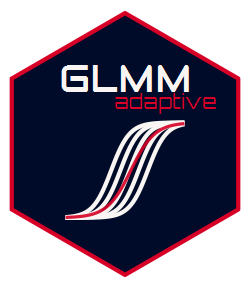## Description

GLMMadaptive fits mixed effects models for grouped/clustered outcome variables for which the integral over the random effects in the definition of the marginal likelihood cannot be solved analytically. The package approximates these integrals using the adaptive Gauss-Hermite quadrature rule.

Multiple random effects terms can be included for the grouping factor (e.g., random intercepts, random linear slopes, random quadratic slopes), but currently only a single grouping factor is allowed.

## Basic Features

• The package contains a single model-fitting function named mixed_model() with four required arguments, fixed a formula for the fixed effects, random a formula for the random effects, family a family object specifying the type of response variable, and data a data frame containing the variables in the previously mentioned formulas.

• Methods for standard generics are provided, i.e., coef(), fixef(), ranef(), vcov(), logLik(), summary(), anova(), confint(), fitted(), residuals(), predict(), and simulate().

• Negative binomial mixed models can be fitted using the negative.binomial() family object.

• Zero-inflated Poisson and negative binomial models using the zi.poisson() and zi.negative.binomial() family objects.

• Hurdle Poisson and negative binomial models using the hurdle.poisson() and hurdle.negative.binomial() family objects.

• Zero-inflated binomial models using the zi.binomial() family objects.

• Two-part/hurdle mixed models for semi-continuous normal data using the hurdle.lognormal() family object.

• Mixed models for censored normal data using the censored.normal() family object.

• Continuation ratio mixed models for ordinal data using functions cr_setup() and cr_marg_probs().

• Beta and hurdle Beta mixed effects models using beta.fam() and hurdle.beta.fam() family objects.

• Gamma mixed effects models using the Gamma() or Gamma.fam() family object.

• Linear mixed effects models with right and left censored data using the censored.normal() family object.

• Users may also specify their own log-density function for the repeated measurements response variable, and the internal algorithms will take care of the optimization.

• Calculates the marginalized coefficients using the idea of Hedeker et al. (2017) using function marginal_coefs().

• Predictions with confidence interval for constructing effects plots are provided by function effectPlotData().

## Basic Use

Let y denote a grouped/clustered outcome, g denote the grouping factor, and x1 and x2 covariates. A mixed effects model with y as outcome, x1 and x2 as fixed effects, and random intercepts is fitted with the code:

fm <- mixed_model(fixed = y ~ x1 + x2, random = ~ 1 | g, data = DF,
family = poisson())

summary(fm)

In the data argument we provide the data frame DF, which contains the aforementioned variables. In the family argument we specify the distribution of the grouped/clustered outcome conditional on the random effects. To include in the random-effects part intercepts and x1, we update the call to mixed_model() as

gm <- mixed_model(fixed = y ~ x1 + x2, random = ~ x1 | g, data = DF,
family = poisson())

summary(gm)

## Installation

The development version of the package can be installed from GitHub using the devtools package:

devtools::install_github("drizopoulos/GLMMadaptive")

and with vignettes

devtools::install_github("drizopoulos/GLMMadaptive", build_opts = NULL)

Hex-sticker courtesy of Greg Papageorgiou @gr_papageorgiou.